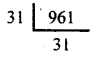# KSEEB Solutions for Class 8 Maths Chapter 5 Squares, Square Roots, Cubes, Cube Roots Ex 5.5

Students can Download Maths Chapter 5 Squares, Square Roots, Cubes, Cube Roots Ex 5.5 Questions and Answers, Notes Pdf, KSEEB Solutions for Class 8 Maths helps you to revise the complete Karnataka State Board Syllabus and score more marks in your examinations.

## Karnataka Board Class 8 Maths Chapter 5 Squares, Square Roots, Cubes, Cube Roots Ex 5.5

Question 1.
Find the nearest integer to the square root of the following numbers.
(i) 232
(ii) 600
(iii) 728
(iv) 824
(v) 1729
Answer:
(i) 232
232 lies between 225 = (15²) and 256 = ( 16²)
232 – 225 = 7 and 256 – 225 = 31
7 < 31
∴ √232 is nearest to 15.

Question (ii)
600
Answer:
600 lies between 5 76 (=24²) and 625 (= 25²)
600 – 576 = 24 and 625 – 600 = 25
24 < 25
∴ √600 is nearest to 24.

Question (iii)
728
Answer:
728 lies between two square
No. 676 and 729
676 = 26 × 26
∴√676 = 26
and 729 = 27 × 27
∴ √729 = 27
Now 728 is nearer to 729.
∴√728 is 27

Question (iv)
824
Answer:
824 lies between 784=(28²) and 841 =(29²)
824 – 784 = 40 and 841 – 824= 17
17 < 40
∴ √824 is nearest to 29.

Question (v)
1729
Answer:
1729 lies between 1681 = (41²) and 1764 = (42²)
1729 – 1681 =48 and 1764- 1729 = 35
35 < 48
∴ √1729 is nearest to 42.

Question 2.
A Piece of land is in the shape of a square and its area is 1000 m². This has to be fenced using barbed wire. The barbed wire is available only integral lengths. What is the minimum length of the barbed wire that has to be bought for this purpose?
Answer:
If l is the side length of a square its perimeter is 4l.
Given that l²= 1000
Hence (4l)²= 16l² = 16 x 1000 = 16000
126² = 15876 < 16000 < 16129= 127²
15876 is nearer to 16000 than 16129. The approximate value of √16000 is 126.
The Approximate Value of the perimeter is 126 m.
If the length of the wire is 126 m it may fall short.
∴ The length of the wire to be bought is 127m.

Question 3.
A student was asked to find V961. He read it wrong and found V691 to the nearest integer. How much small was his number from the correct answer?
Answer:
√961 = 31 is the correct answer 3126² = 676 < 691 <729 =27²
691 – 676 = 15 and 729 – 691 =38
√691 is nearer to 26
The student √691 = 26 found. The correct answer is 31.
∴ The difference is 31 – 26 = 5
The number is smaller than the correct answer by 5.